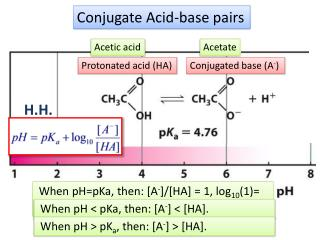DownloadDownload PresentationConjugate Acid-base pairs

# Conjugate Acid-base pairs

Download Presentation## Conjugate Acid-base pairs

- - - - - - - - - - - - - - - - - - - - - - - - - - - E N D - - - - - - - - - - - - - - - - - - - - - - - - - - -
##### Presentation Transcript

1. Conjugate Acid-base pairs Acetic acid Acetate Protonated acid (HA) Conjugated base (A-) H.H. When pH=pKa, then: [A-]/[HA] = 1, log10(1)= 0. When pH < pKa, then: [A-] < [HA]. When pH > pKa, then: [A-] > [HA].

2. Buffer = HA + A- X If pKa = 2.5, then pH=? Log101 = 0 [Illinic acid buffer/Illinate buffer] Illinate Illinic acid 1 M 1 M + [illinate (A-)] [illinic acid (HA)]

3. Q11a. What is the concentration of a lactic acid buffer (pKa = 3.9) that contains 2.701 M of CH3CH(OH)COOH and 0.901 M of CH3CH(OH)COO-? Give your answer using 2 decimal points . [Buffer] = [HA] + [A-] = 2.701 M + 0.901M ANSWER: Final Concentration of lactic acid buffer = 3.60 M b) What is the pH? Give your answer using 1 decimal point. pH = pKa + log10 [A-]/[HA] = 3.9 + log10 (0.901 M/2.701M) = 3.9 + log10 (0.34) = 3.9 + (-0.5) ANSWER: pH = 3.4 H.H.

4. Q13. A molecule has pKa values of 7, 8, and 9. At pH equals 7.0, estimate roughly what is the deprotonated state of the three groups (expressed as %), respectively? Use 2 decimal points. Do not use scientific notation to enter your answer. pH = pKa + log10 ([A-]/[HA]) Group 7: 7.0 – 7.0 = log10 ([A-]/[HA]) = 0 100=([A-]/[HA])=1 (ratio; 1 part A- and 1 part HA), therefore A- is 1 part out of 2 parts Answer: is 50.00 % Group 8 7.0 – 8.0 = log10 ([A-]/[HA]) = -1 10-1=([A-]/[HA])=0.1 (1 part A- and 10 part HA) --------- 11 parts total, therefore 1/11 x 100 = Answer: is 9.09 % H.H.

5. 12a. A 500 mM solution of illinic buffer containing 100 mM of illinate (conjugate base) has a pH of 3.3. Calculate the Ka of illinic acid. [Buffer] = [HA] + [A-] [HA] = 500 mM -100 mM = 400 mM pH = pKa + log10 ([A-]/[HA]) pKa = pH - log10 ([A-]/[HA]) = 3.3-log10 (100mM/400mM)=3.3 – (-0.6)=3.9 pKa = -log10 Ka or Ka = 10-pKa =10-3.9 ANSWER: Ka of illinic acid = 1.26×10-4 M Can get from getting pKa H.H. b) How much (in mL) of 5 M solution of NaOH (strong base) would be needed to bring 1 L of the above illinic buffer to a pH of 4.3?

6. Buffer = HA + A- OH H+ = H2O + Acetate Acetic acid 1.90 M 1 M 1 M 0.1 M + [acetate (A-)] [acetic acid (HA)] 2 M

7. 12a. A 500 mM solution of illinic buffer containing 100 mM of illinate (conjugate base) has a pH of 3.3. Calculate the Ka of illinic acid. ANSWER: Ka of illinic acid = 1.26×10-4 M (pKa = 3.9) 12b. How much (in mL) of 5 M solution of NaOH (strong base) would be needed to bring 1 L of the above illinic buffer to a pH of 4.3? pH = pKa + log10 ([A-]/[HA]) 4.3 – 3.9 = log10 ([A-]/[HA]) 0.4 = log10 ([A-]/[HA]) At pH 4.3, the ratio is 100.4 = ([A-]/[HA]) = 2.5 (same as 5 parts A- and 2 parts HA). A- is 5 out 7 parts or 71% (355 mM) At pH 3.3 (from part A), ratio is 0.25, where A- is 1/5 of buffer or 20% (100 mM out of 500 mM) To go from 100 mmoles to 355 mmoles requires 255 mmoles of NaOH @ 5 mmoles/mL Is:ANSWER: 51.0 mL H.H.

8. How to make acetic acid/acetate buffer Option 1: Add acetic acid solution (HA) and acetate solution (A-) at a ratio giving you the desired pH Option 2: Start with a solution of acetic acid solution (HA) and add strong base (NaOH) until you reach desired pH Option 3: Start with acetate solution (A-) and add strong acid (HCl) until you reach the desired pH